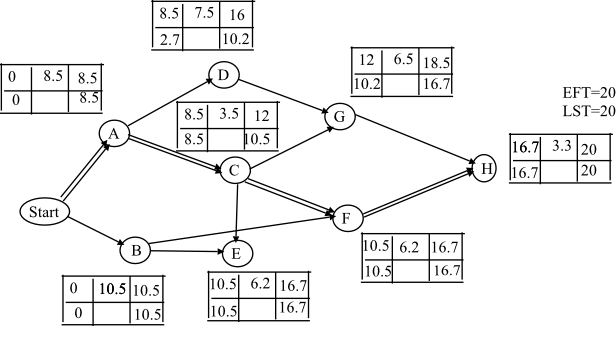# Answered! draw the AON diagram; find the critical path and the expected project completion time. Then compute the…

draw the AON diagram; find the critical path and the expected project completion time. Then compute the probability of completing the project in (i) 21 days; (ii) 22 days; and (iii) 25 days.

Expected time – (optimistic + 4x monthly + Pessimistic) / 6

Don't use plagiarized sources. Get Your Custom Essay on
Answered! draw the AON diagram; find the critical path and the expected project completion time. Then compute the…
GET AN ESSAY WRITTEN FOR YOU FROM AS LOW AS \$13/PAGE
 Task a m b Expected Variance Std Dev. A 6.5 7.5 14.5 8.5 1.77 1.33 B 8.5 10.5 12.5 10.5 .45 .67 C 2.5 3.5 4.5 3.5 .11 .33 D 6.5 7.5 8.5 7.5 .11 .33 E 5.5 5.5 9.5 6.2 .45 .67 F 5.5 7.5 9.5 7.5 .45 .67 G 4.5 6.5 8.5 6.5 .45 .67 H 2.5 3.5 3.5 3.3 .03 .17
 Activity Predecessors Start None A Start B Start C A D A E B, C F B, C G D, E H F End G, H

AON (Activity-on-Node) Diagram:

AON diagram is used to estimate the project starting date and finishing date, and also determines the critical path.

Three estimation times are used for each activity to determine the time required to complete the project, which are Optimistic time (a), pessimistic time (b), most likely time (m).

Expected activity time (t) = (a+4m+b)/6

Variance = [(b-a)/6]2

Standard deviation = square root (variance)

The formulas used to determine the critical path are shown below:

Earliest start time (EST): Max (Earliest finishing time of all immediate predecessor)

Earliest finishing time (EFT): Earliest starting time + activity time

Latest start time (LST): Latest finishing time + activity time

Latest finishing time (LFT): Min (Latest finish time of all following activities)

The estimated activity time and standard deviation and variance or shown in the table. Therefore, construct the AON diagram with the estimated activity time as shown below:Note: 1st row is EST and 2nd is activity time and 3rd is EFT and LST and LFT are reverse.

Calculation for EST and EFT:

Calculation for EST and EFT for activity A is shown below:

• EST is 0 because it is the starting activity.
• EFT is 8.5 given in the table.

Calculation for EST and EFT for activity B is shown below:

• EST is 8.5 because it is started after completion of A.
• EFT is 10.2 because 8.5 + 7.5 = 10.2.

Calculation for EST and EFT for activity B is shown below:

• EST is 0 because it is starting activity.
• EFT is 10.5 because 10.5 + 0 = 10.5.

EST and EFT for all activities are calculated in similar way which is shown in the diagram. If the activity starts from the completion of two activities, consider the minimum EFT of the predecessor.

Activity G starts after C and D, but, the EFT of c is less than the EFT of D. therefore, C is the EST of G.

Calculation of LST and LFT:

• LST is the backward calculation from H. It is 0 and LFT is 16.7.
• If an activity is comes from two activities, consider the maximum LFT of the follower.

Activity C is comes from G, F and E but F and E are same (10.5) is greater than G. therefore EST of C is 10.5.

Finally LFT and EST are 0.

From the calculation, the minimum duration to complete the project is 20 days. And the critical path if A-C-F-H which is represented by double line in the diagram.

Therefore, the project completion time = 20 days

• Probability to complete the project for 21 days is calculated as shown below:

Probability to complete the project = (Target time-expected time)/ standard deviation

= (21-20) / (adding of all CPM standard deviations)

= (1) / 2.5 = 0.4

Probability to complete the project for 21 days is 0.4

• Probability to complete the project for 22 days is 0.8
• Probability to complete the project for 23 days is 2.5